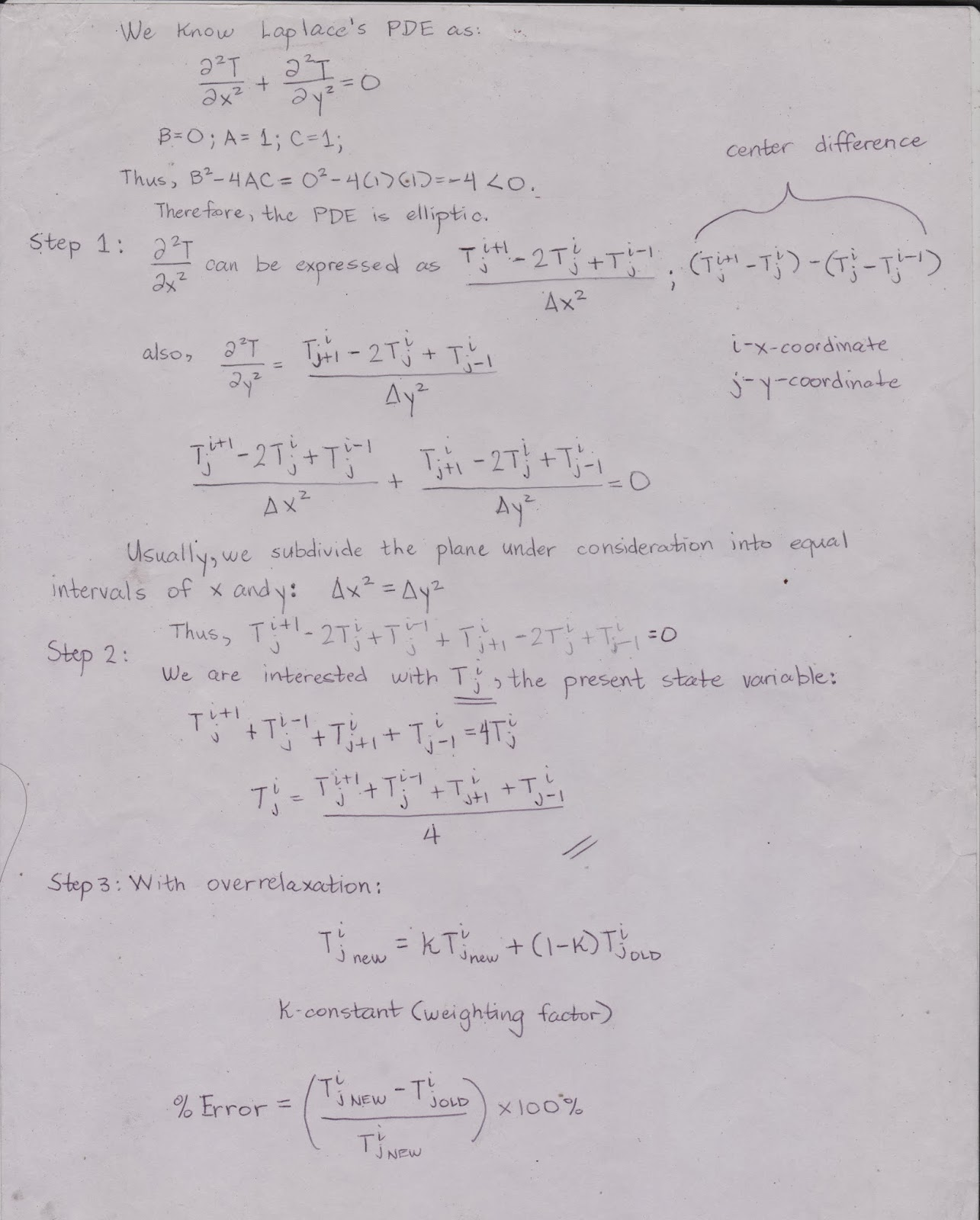Liebmann Method

This is simply the Gauss-Seidel method applied for elliptic PDEs (i.e. B^2-4*A*C<0, usually steady-state).

A good example would be applications of the Laplace PDE to heat conduction.

(Working with Maths symbols is hard with a keyboard so I'll just do it on paper)General steps in using numerical methods for solving PDEs:

Step 1: Transform the continuous PDE into a discrete representation.

Step 2: Isolate the present state variable (or the variable asked by the problem).

Step 3: Use overrelaxation if necessary to fasten convergence.Explicit Method

This method is used for parabolic PDEs with a little problem in stability. (i.e. B^2-4*A*C=0).

A good example would be applications of the Fourier's Law of Heat Conduction.The problem above differs from the plate problem due to the fact that we are dealing with unbounded sides. Also, the explicit method is only stable when the "constant" (that is, 0.02087 we've used in the problem) is less than 1/2.